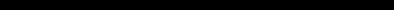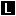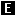# SINCLAIR ZX SPECTRUM BASIC ProgrammingCHAPTER 9
Functions

#### Summary

DEF, LEN, STR\$. VAL, SGN. ABS, INT, SQR, FN

Consider the sausage machine. You put a lump of meat in at one end, turn a handle, and out comes a sausage at the other end. A lump of pork gives a pork sausage, a lump of fish gives a fish sausage, and a load of beef a beef sausage.

Functions are practically indistinguishable from sausage machines but there is a difference: they work on numbers and strings instead of meat. You supply one value (called the argument), mince it up by doing some calculations on it, and eventually get another value, the result.

 Meat in -> Sausage Machine -> Sausage out Argument in -> Function -> Result out

Different arguments give different results, and if the argument is completely inappropriate the function will stop and give an error report.

Just as you can have different machines to make different products - one for sausages. another for dish cloths, and a third for fish-fingers and so on, different functions will do different calculations. Each will have its own value to distinguish it from the others.

You use a function in expressions by typing its name followed by the argument, and when the expression is evaluated the result of the function will be worked out.

As an example, there is a function called LEN, which works out the length of a string. Its argument is the string whose length you want to find, and its result is the length, so that if you type

PRINT LEN "Sinclair"

the computer will write the answer 8. the number of letters in 'Sinclair'. (To get LEN, as with most function names, you must use extended mode: press CAPS SHIFT and SYMBOL SHIFT at the same time to change the cursor fromto, and then press the K key.)

If you mix functions and operations in a single expression, then the functions will be worked out before the operations Again, however, you can circumvent this rule by using brackets. For instance, here are two expressions which differ only in the brackets, and yet the calculations are performed in an entirely different order in each case (although, as it happens, the end results are the same).

 LEN "Fred"+ LEN "Bloggs" LEN ("Fred"+"Bloggs") 4+LEN "Bloggs" LEN ("FredBloggs") 4+6 LEN "FredBloggs" 10 10

Here are some more functions.

STR\$ converts numbers into strings: its argument is a number, and its result is the string that would appear on the screen if the number were displayed by a PRINT statement. Note how its name ends in a \$ sign to show that its result is a string. For example, you could say

LET a\$=STR\$ 1e2

which would have exactly the same effect as typing

LET a\$="100"

Or you could say

PRINT LEN STR\$ 100,000

and get the answer 3, because STR\$ 100.0000="100".

VAL is like STR\$ in reverse: it converts strings into numbers. For instance,

VAL "3.5"=3.5

In a sense, VAL is the reverse of STR\$, because if you take any number, apply STR\$ to it, and then apply VAL to it, you get back to the number you first thought of.

However, if you take a string, apply VAL to it, and then apply STR\$ to it, you do not always get back to your original string.

VAL is an extremely powerful function, because the string which is its argument is not restricted to looking like a plain number - it can be any numeric expression. Thus, for instance,

VAL "2*3"=6

or even,

VAL ("2"+"3") = 6

There are two processes at work here. In the first, the argument of VAL is evaluated as a string: the string expression "2"+"*3" is evaluated to give the string "2*3". Then, the string has its double quotes stripped off, and what is left is evaluated as a number: so 2*3 is evaluated to give the number 6.

This can get pretty confusing if you don't keep your wits about you; for instance,

PRINT VAL "VAL""VAL""""2"""""""

(Remember that inside a string a string quote must be written twice. If you go down into further depths of strings, then you find that string quotes need to be quadrupled or even octupled.)

There is another function, rather similar to VAL, although probably less useful, called VAL\$. Its argument is still a string, but its result is also a string. To see how this works, recall how VAL goes in two steps: first its argument is evaluated as a string, then the string quotes stripped off this, and whatever is left is evaluated as a number. With VAL\$, the first step is the same, but after the string quotes have been stripped off in the second step, whatever is left is evaluated as another string. Thus

VAL\$ """Fruit punch""" = "Fruit punch"
(Notice how the string quotes proliferate again.) Do

LET a\$="99"

and print out all of the following: VAL a\$, VAL "a\$", VAL """a\$""", VAL\$ a\$, VAL\$ "a\$" and VAL\$ """a\$""". Some of these will work, and some of them won't; try to explain all the answers. (Keep a cool head.)

SGN is the sign function (sometimes called signum). It is the first function you have seen that has nothing to do with strings, because both its argument and its result are numbers. The result is +1 if the argument is positive, 0 if the argument is zero, and -1 if the argument is negative.

ABS is another function whose argument and result are both numbers. It converts the argument into a positive number (which is the result) by forgetting the sign, so that for instance

ABS -3.2 = ABS 3.2 = 3.2

INT stands for 'integer part' - an integer is a whole number, possibly negative. This function converts a fractional number into an integer by throwing away the fractional part, so that for instance,

INT 3.9=3

Be careful when you are applying it to negative numbers, because it always rounds down: thus, for instance,

INT -3.9=-4

SQR calculates the square root of a number - the result that, when multiplied by itself, gives the argument. For instance,

SQR 4 = 2 because 2*2=4
SQR 0.25 = 0.5 because 0.5*0.5=0.25
SQR 2 = 1.4142136 (approximately) because 1.4142136*1.4142136=2.0000001

If you multiply any number (even a negative one) by itself, the answer is always positive. This means that negative numbers do not have square roots, so if you apply SQR to a negative argument you get an error report A Invalid Argument.

You can also define functions of you own. Possible names for these are FN followed by a letter (if the result is a number) or FN followed by a letter followed by \$ (if the result is a string). These are much stricter about brackets: the argument must be enclosed in brackets.

You define a function by putting a DEF statement somewhere in the program. For instance, here is the definition of a function FN s whose result is the square of the argument:

10 DEF FN s(x)=x*x: REM the square of x

DEF is obtained in extended mode, using SYMBOL SHIFT and 1. When you type this, the computer will give you FN automatically, because in a DEF statement the DEF is always followed immediately by FN. After this, the s completes the name FN s of the function.

The x in brackets is a name by which you wish to refer to the argument of the function. You can use any single letter you like for this (or, if the argument is a string, a single letter followed by \$).

After the = sign comes the actual definition of the function. This can be any expression, and it can also refer to the argument using the name you've given it (in this case, x) as though it were an ordinary variable.

When you have entered this line, you can invoke the function just like one of the computer's own functions, by typing its name, FN s, followed by the argument. Remember that when you have defined a function yourself, the argument must be enclosed in brackets. Try it out a few times:

PRINT FN s(2)
PRINT FN s(3+4)
PRINT 1+1NT FN s (LEN "chicken"/2+3)

Once you have put the corresponding DEF statement into the program, you can use your own functions in expressions just as freely as you can use the computer's.

Note: in some dialects of BASIC you must even enclose the argument of one of the computer's functions in brackets. This is not the case in ZX Spectrum BASIC.

INT always rounds down. To round to the nearest integer, add .5 first - you could write your own function to do this.

20 DEF FN r(x)=lNT (x+O.5): REM gives x rounded to the nearest integer.

You will then get, for instance,

 FN r(2.9) = 3 FN r(2.4) = 2 FN r(-2.9) = -3 FN r(-2.4) = -2

Compare these with the answers you get when you use INT instead of FN r. Type in and run the following:

10 LET x=0: LET y=0: LET a=10
20 DEF FN p(x,y)=a+x
30 DEF FN q()=a+x*y
40 PRINT FN p(2,3),FN q()

There are a lot of subtle points in this program.

First, a function is not restricted to just one argument: it can have more, or even none at all - but you must still always keep the brackets.

Second, it doesn't matter whereabouts in the program you put the DEF statements. After the computer has executed line 10, it simply skips over lines 20 and 30 to get to line 40. They do, however, have to be somewhere in the program. They can't be in a command.

Third, x and y are both the names of variables in the program as a whole, and the names of arguments for the function F p. FN p temporarily forgets about the variables called x and y, but since it has no argument called a, it still remembers the variable a. Thus when FN p(2,3) is being evaluated, a has the value 10 because it is the variable, x has the value 2 because it is the first argument, and y has the value 3 because it is the second argument. The result is then, 10+2*3=16. When FN q() is beinq evaluated, on the other hand. there are no arauments. so a. x and v all still refer to the variables and have values 10, 0 and 0 respectively. The answer in this case is 10+0*0=10.

Now change line 20 to

20 DEF FN p(x,y)=FN q()

This time, FN p(2,3) will have the value 10 because FN q will still go back to the variables x and y rather than using the arguments of FN p.

Some BASlCs (not the ZX Spectrum BASIC) have functions called LEFT\$, RIGHT\$, MID\$ and TL\$.

LEFT\$ (a\$,n) gives the substring of a\$ consisting of the first n characters.

RIGHT\$ (a\$,n) gives the substring of a\$ consisting of the characters from nth on.

MID\$ (a\$, n1, n2) gives the substring of a\$ consisting of n2 characters starting at the n1th.

TL\$ (a\$) gives the substring of a\$ consisting of all its characters except the first.

You can write some user-defined functions to do the same: e.g.

10 DEF FN t\$(a\$)=a\$(2 TO ): REM TL\$
20 DEF FN I\$(a\$ n)=a\$( TO n): REM LEFT\$

Check that these work with strings of length 0 or 1.

Note that our FN I\$ has two arguments, one a number and the other a string. A function can have up to 26 numeric arguments (why 26?) and at the same time up to 26 string arguments.

#### Exercise

Use the function FN s(x)=x*x to test SQR: you should find that

FN s(SQR x)=x

if you substitute any positive number for x, and

SQR FN s(x)=ABS x

whether x is positive or negative (Why the ABS?).